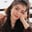Related Tags

python programming
communitycreator

# What is cmath.polar(x) in Python?Fouzia Bashir

The cmath.polar(x) method returns a demonstration of the argument x in polar coordinates.

We can pass int, float, and complex type values.

In polar coordinates, a complex number is defined by a modulus and phase angle pair, i.e., (r, phi).

The cmath module in Python is used to deal specifically with complex numbers.

### Syntax

cmath.polar(x)


### Parameters

This method accepts the single arguments of int, float, and complex types.

• x: The number to find polar coordinates.

### Return value

This method returns a tuple (r,phi), where r is the modulus of x and phi is a phase angle.

• r: The first value in a two-value tuple. Represents the modulus.

• phi: The second value in a two-value tuple. Represents the phase.

### Code

In the code snippet below, we have positive, negative, and complex numbers as an argument.

This will result in polar coordinates as a tuple of the modulus and phase angle (r,phi).

import cmath
# Positive x
print(cmath.polar(90.0))
# Negative x
print(cmath.polar(-90.0))
# Complex number
print (cmath.polar(3 + 2j))

RELATED TAGS

python programming
communitycreator

CONTRIBUTORFouzia Bashir
RELATED COURSES

View all Courses

Keep Exploring

Learn in-demand tech skills in half the time